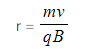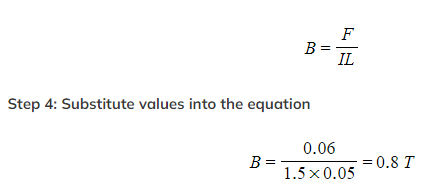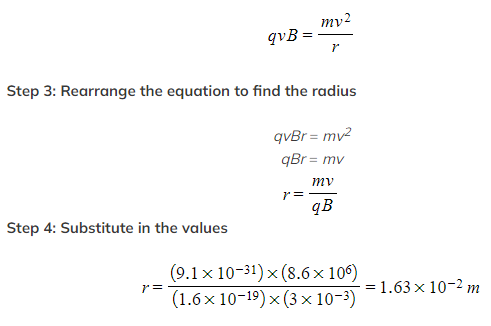# IB DP Physics: HL复习笔记5.4.4 Solving Problems Involving Magnetic Forces

### Solving Problems Involving Magnetic Forces

• There is a lot of mathematics surrounding magnetism and magnetic forces
• The key equations are:
Force on a current-carrying conductor:
F = BIL

Force on a moving charge:
F = Bqv

Radius of a moving charge in a magnetic field:• Below are two worked examples demonstrating different situations involving magnetic forces

#### Worked Example

A 5 cm length of wire is at 90° to the direction of an external magnetic field. When a current of 1.5 A flows through the wire it experiences a force of 0.06 N from the motor effect.

Calculate the magnetic flux density of the magnet.

Step 1: List the known quantities
• Length, L = 5 cm = 0.05 m
• Current, I = 1.5 A
• Force, F = 0.06 N
Step 2: Write out the equation for magnetic force
F = BIL
Step 3: Rearrange the equation to make B the subject#### Worked Example

This question is about the movement of an electrically charged particle into a magnetic field. An electron enters a magnetic field and moves in an approximately circular path as shown below.1. Explain whether the speed of the proton is the same when entering and exiting the magnetic field.
2. The magnetic field has a strength of 0.3 T and the velocity of the electron before entering the magnetic field is 8.6 × 106 m s−1 to the left. Show that the radius of the motion of the electrons is 1.63 cm.
Part (a)
• The answer is that the work done by the magnetic force on the charge must be zero
• This is because the force itself is at right angles to the velocity
• Since the work done is zero, therefore the kinetic energy does not change between entering and leaving the magnetic field
• Therefore the speed is the same
Part (b)
Step 1: List the known quantities
• Magnetic field strength, B = 0.3 T
• Velocity of electron (before entering field), v = 8.6 × 106 m s−1 to the left
• Radius of motion to be shown, r = 1.63 cm
Step 2: Equate the magnetic force and the force of circular motion
• This can be done for the situation since the circular motion is caused by the magnetic forceStep 5: State final answer
R = 1.63 × 10−2 m = 1.63 cm in a circular motion